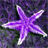QlikView App Dev

Discussion Board for collaboration related to QlikView App Development.

Announcements
QlikWorld 2022, LIVE in Denver CO., May 16-19, 2022. REGISTER NOW TO RECEIVE EARLY BIRD PRICING
cancel
Showing results for
Did you mean:Contributor II

How to get 'Sum of Rows' in Text box instead of 'Expression Total' ?

Hi all,

I have a Text Box to display the value for an expression like sum(A)*B, for any number of dimensions selected by the user.

By default it is showing 'Expression Total', which is not giving correct results because of multiplication involved.

I need to show 'Sum of the Rows' in the same.

Data example :

 Dimension A B D1 10 2 D2 12 3 D3 13 4

If D1, D2 and D3 are selected,

Expression Total:   (10+12+13) * (2+3+4) = 35 * 9 = 315 (incorrect)

Sum of Total: (10*2)+(12*3)+(13*4) = 108 (correct)

Can anyone suggest a solution?

1 Solution

Accepted SolutionsPartner

Hi Jaspreet Sran

maybe you could use aggr() function like this :

aggr(sum(A*B),Dimension)

3 RepliesPartner

Hi Jaspreet Sran

maybe you could use aggr() function like this :

aggr(sum(A*B),Dimension)Creator III

try sum(A*B)Contributor II
Author

Hi Luc,

yes, you are right, just need to add sum outside this to get sum for all the dimensions.

sum(aggr(sum(A*B),Dimension))

Thanks,

Jaspreet Kaur SranCommunity Browser